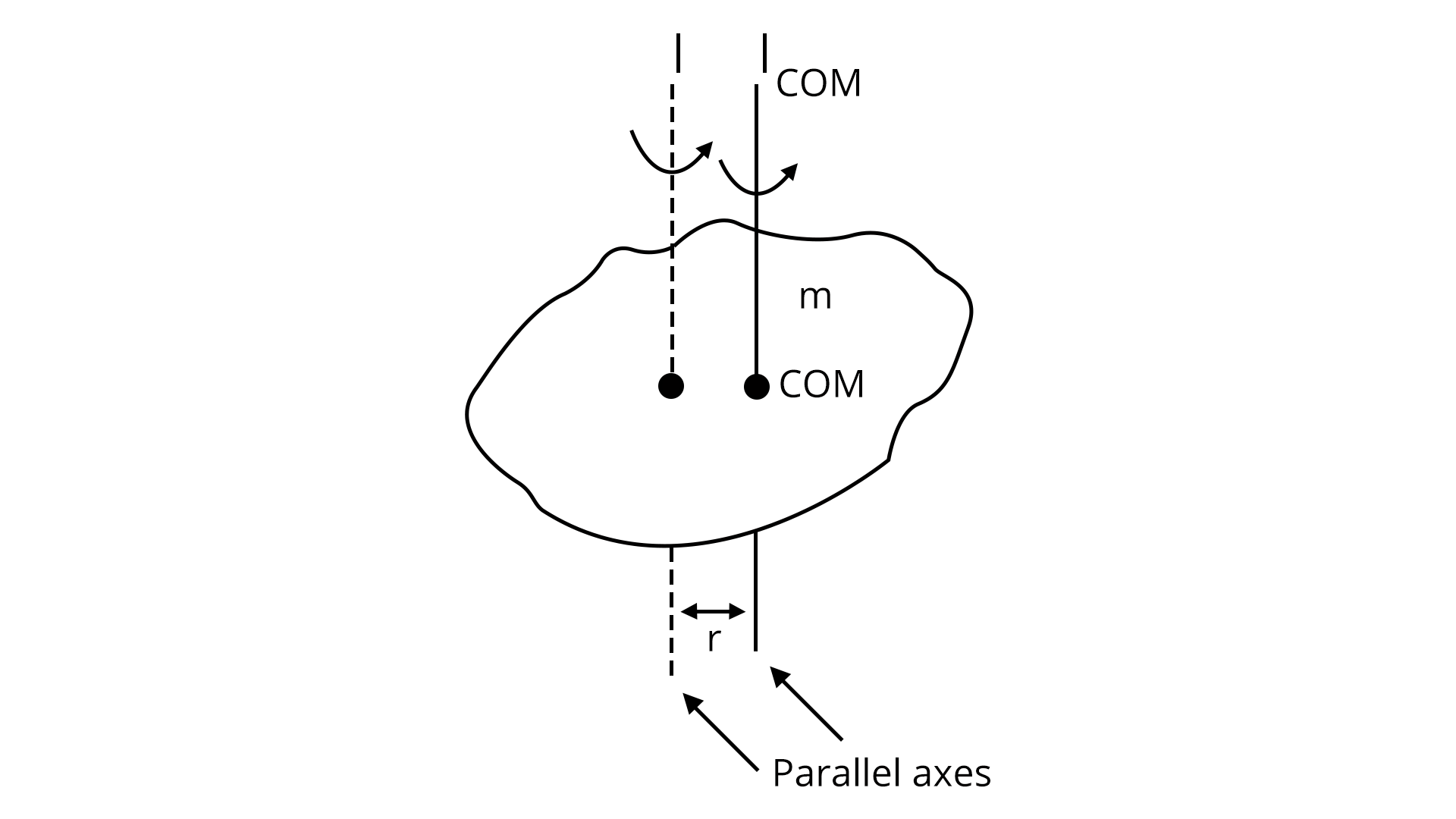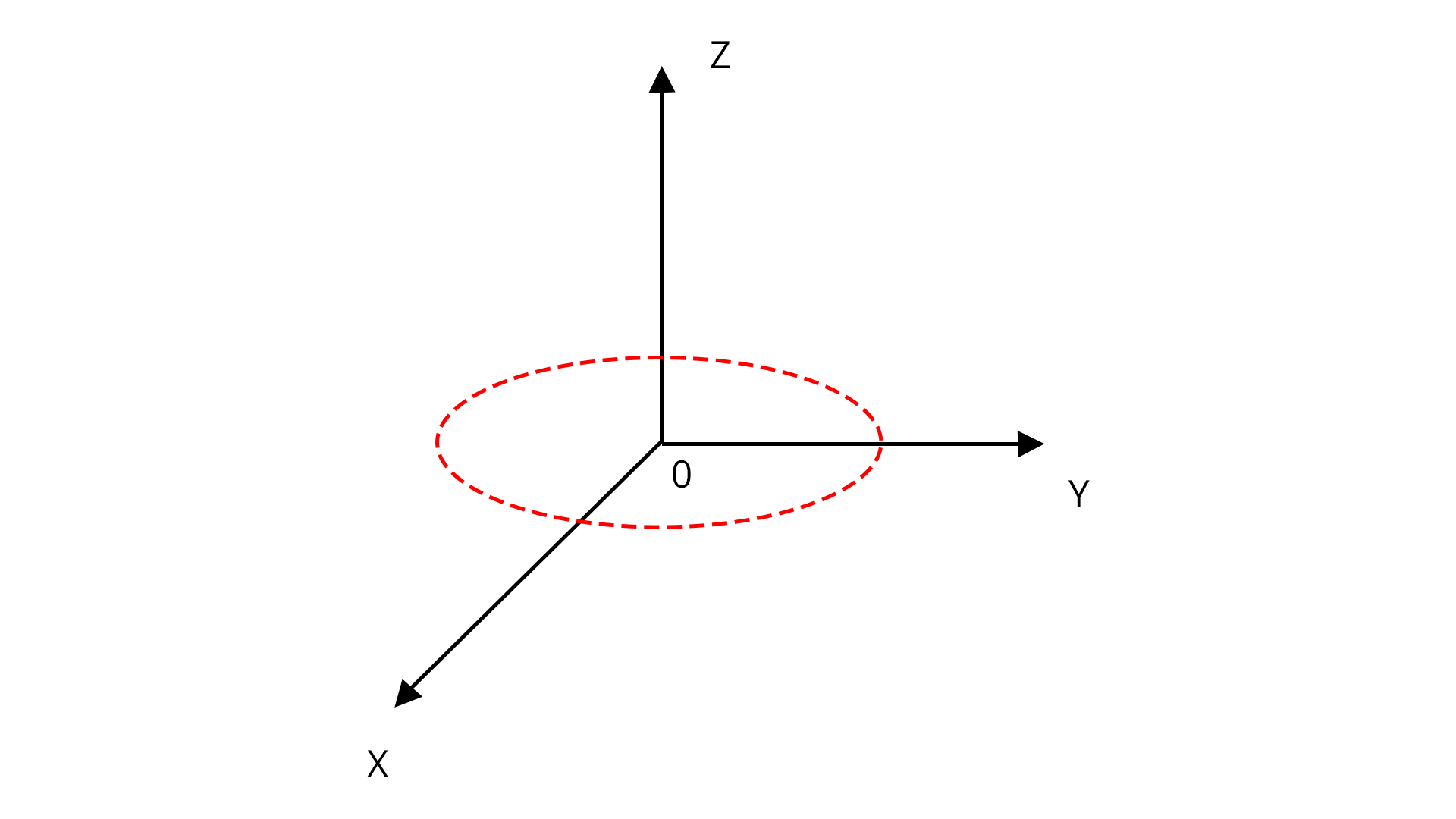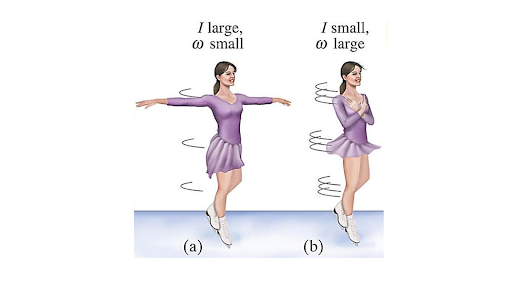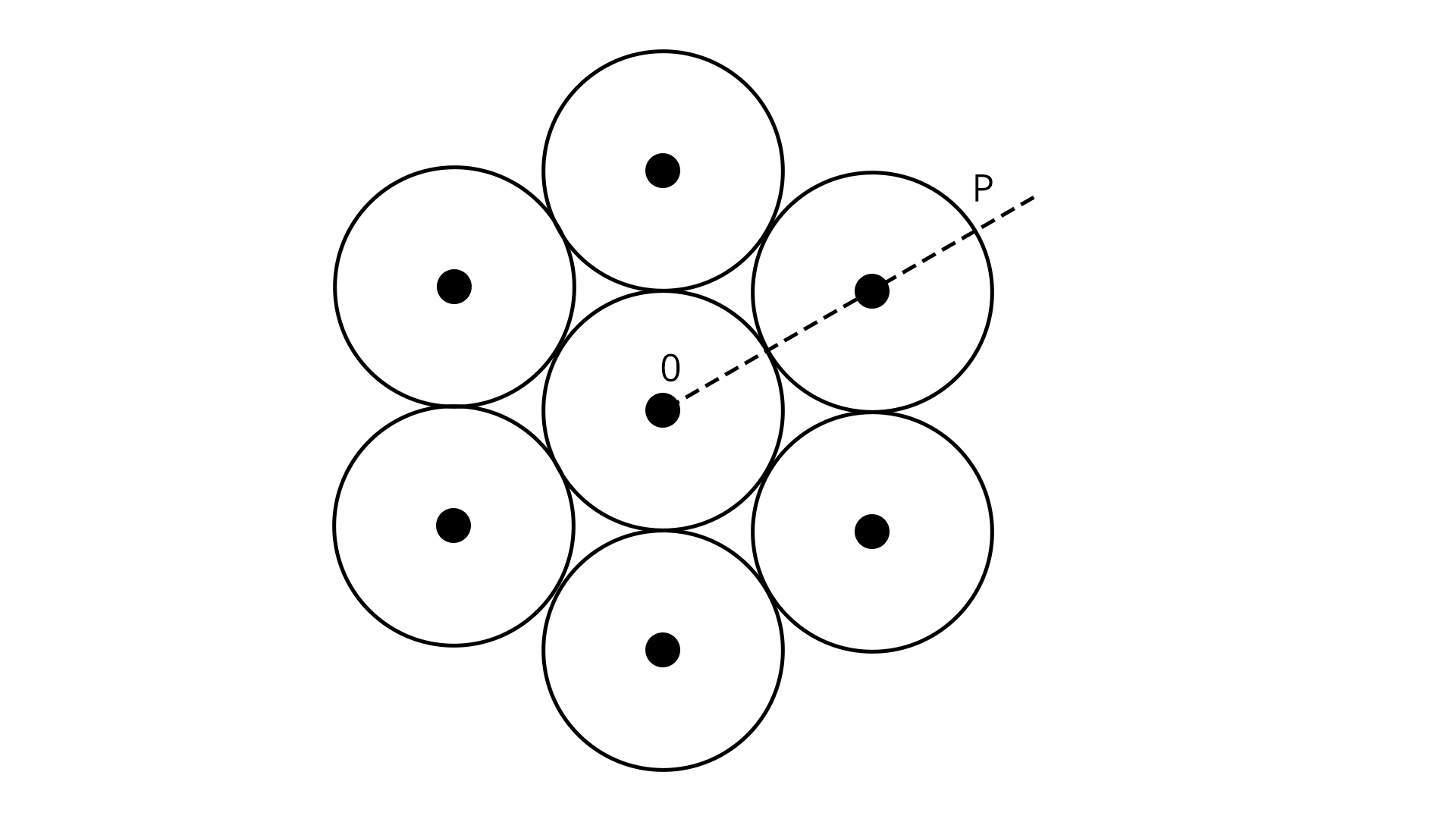Courses
Courses for Kids
Free study material
Free LIVE classes
More

# JEE Important Chapter - Rotational Motion

Get interactive courses taught by top teachers## Rotational Motion of Objects

Last updated date: 27th May 2023
Total views: 117k
Views today: 10.50k

The rotational motion of the objects plays an important role in understanding dynamics in 3D. In the past chapters, students have learned about the motion in one and two-dimensional planes, where an object will be in a straight line motion. Now the question arises will the same kinematics theory and equations be applicable if the object starts rolling? To answer this question students should study the chapter on rotational motion and understand what is rotational motion.

The rotational motion chapter deals with all the phenomena and theories related to rotation and rotational motion examples. In the rotational motion chapter, we will look at the kinematics and dynamics of a solid body in two different motions. Rotation around a stationary axis, often known as pure rotation, is the first type of motion for a solid body. The second type of solid motion is plane motion, in which the solid body's centre of mass moves in a fixed direction while the body's angular velocity remains perpendicular to that plane at all times.

Furthermore, in the rotational motion chapter, we study the rotational dynamics and the parameters associated with it. The chapter includes many important concepts such as angular velocity, angular acceleration, perpendicular axis theorem, parallel axis theorem, and torque, and we define the moment of inertia of various objects such as a ring, disc, cylinder, etc.. which are all important in exam perspective.

In this article, we will cover the concepts which are useful for the JEE exam and boost their exam preparation.

## JEE Main Physics Chapter-wise Solutions 2022-23

### Important Topics of Rotational Motion

• Rigid Bodies

• Rotational Kinematics

• Moment of Inertia

• Parallel Axis Theorem

• Perpendicular Axis Theorem

• Torque

• Angular Momentum

• Angular Displacement

• Conservation of Angular Momentum

• Instantaneous Axis of Rotation

• Rolling motion

• Toppling

### Important Concepts of Rotational Motion

 Sl. No Name of Concept Key Points To Remember 1. Rigid Bodies A rigid body is basically a solid body in which the shape and size of the body remain unchanged under motion and interaction with other bodies. 2. Motion of a Rigid Body Rigid bodies undergo mainly three types of motion:1. Pure Rotational: The rotation of the body about a fixed axis is known as rotational motion. Pure rotational motion means that all the points at the same radius from the centre of rotation will have the same velocity.Pure rotational motion examples or rotary motion examples: Rotation of the ceiling fans2. Pure Translational: A rigid body is said to be in pure translational motion if any straight line fixed to it remains parallel to its initial orientation all the time.Ex: A bike/car moving along a straight long road3. Translational+Rotational: When a rigid body executes pure rotation around an axis while also translational motion with respect to a reference frame, it is said to be in combined translational and rotational motion.Ex: A rolling wheel 3. Rotational Kinematics Just like in linear kinematics, in rotational kinematics we study the parameters related to rotational motion. Such as angular velocity, angular displacement, angular acceleration, etc.. 4. Moment of Inertia Moment of Inertia plays an important role in the study of rotational dynamics. It is defined as:$I=\displaystyle\sum\limits_{i=1}^n {m_i}{r_i}^2$Where, ri- The perpendicular distance of ith particle of mass mi from the axis of rotationFor a continuous body moment of inertia is defined as:$I=\int {r^2} dm$ Where,dm- Mass of an infinitesimal element of the body at a perpendicular distance r from the axis of rotationNote: Moment of inertia does not depend on the mass of the body 5. Centre of the Mass of a Rigid Body Centre of the mass of a rigid body is an imaginary point where the mass of the entire system is concentrated with external forces focusing on this balancing point. 6. Parallel Axis Theorem Statement: The Moment of Inertia of a body about any axis is equal to the sum of the Moment of Inertia of the body about a parallel axis passing through its centre of mass and the product of its mass and the square of the distance between the two parallel axes.$I={I_C}+M {d^2}$Where,d- The perpendicular distance between the two axes. 7. Perpendicular Axis Theorem Statement:The moment of Inertia of a planar body about an axis perpendicular to its plane is equal to the sum of its moments of inertia about two perpendicular axes concurrent with a perpendicular axis and lying in the plane of the body.${I_Z}={I_X}+{I_Y}$ 8. Conservation of Angular Momentum Statement:When the resultant torque acting on a system of particles is zero, then the total angular momentum vector of the system remains constant.I.e., $\vec\tau_{net}=0$### List of Important Formulae

 Sl. No. Name of Concept Formula 1. Moment of Inertia of circular-shaped bodies Ring: $I= M {R^2}$Disc : $I=\dfrac { M{R^2}}{2}$Solid sphere: $I=\dfrac {2}{5} M {R^2}$Spherical shell: $I=\dfrac {2}{3} M{R^2}$ 2. Moment of inertia of cylindrical bodies Hallow cylinder: $I= M {R^2}$Solid cylinder: $I=\dfrac { M{R^2}}{2}$Thick-walled cylinder: $I=\dfrac {M \left ({R_1}^2+{R_2}^2\right )}{2}$Solid cylinder of length l: $I=\dfrac {M{R^2}}{4} +\dfrac {M {l^2}}{12}$ 3. Moment of inertia of a thin rod Thin rod of length l: $I=\dfrac {M{l^2}}{12}$Special case:If axis of rotation is Perpendicular to rod at one end.$I=\dfrac {M{l^2}}{3}$ 4. Moment of inertia of a rectangular sheet $I=\dfrac {M \left ({l^2}+{b^2}\right )}{2}$ 5. Torque $\tau =\vec r \times \vec F$ 6. Radius of gyration $K=\sqrt {\dfrac {I}{M}}$ 7. Rotational work and energy Rotational work: $W_{rot}=\int \tau d\theta$Kinetic energy: $k_{rot}=\dfrac {1}{2} I {\omega^2}$ 8. Angular momentum $L=\vec r \times \vec P$ 9. Angular momentum of a rigid body in combined translational and rotational motion $\vec L=\vec {L_{cm}}+M\left ( \vec {r_o}+\vec {v_o}\right )$ 10. Angular impulse $\vec J=\Delta \vec L$

### Important Comparison of Rotational Kinematics and Linear Kinematics

When we start preparing for our exams its essential to know our understanding with the help of comparisions, differences between the concepts we have previously studied. We know the kinematics of linear motion, now let us have a look at the comparison of linear kinematics with rotational motion.

 Rotational Kinematics Linear Kinematics Linear position is denoted by S Angular position is denoted by $\theta$ Displacement: $\Delta S= {S_2}- {S_1}$ Angular Displacement: $\Delta {\theta}={\theta_2}-{\theta_1}$ Average velocity: $v_{avg}=\dfrac {\Delta S}{\Delta t}$ Angular average velocity: $\omega_{avg}=\dfrac {\Delta \theta}{\Delta t}$ Instantaneous velocity: $v_{ins}= \lim_{{\Delta t} \rightarrow 0} \dfrac {\Delta S}{\Delta t}$ Instantaneous angular velocity: ${\omega_{ins}}=\lim_{{\Delta t} \rightarrow 0} \dfrac {\Delta \theta}{\Delta t}$ Average acceleration: $a_{avg}=\dfrac {\Delta v}{\Delta t}$ Average angular acceleration: ${\alpha_{avg}}=\dfrac {\Delta \omega}{\Delta t}$ Instantaneous acceleration:$a_{ins}=\lim_{{\Delta t} \rightarrow 0} \dfrac {\Delta v}{\Delta t}$ Instantaneous angular acceleration:${\alpha_{ins}}=\lim_{{\Delta t} \rightarrow 0} \dfrac {\Delta \omega}{\Delta t}$ Linear equations of motion:$v={v_o}+at$${v^2}={{v_o}^2}+2as$$s={v_o}t+\dfrac {1}{2} a{t^2}$ Rotational equations of motion:$\omega = {\omega_o}+\alpha t$${\omega^2}={\omega_o}^2+2 \alpha \theta$$\theta={\omega_o}t+\dfrac {1}{2} \alpha {t^2}$

### Solved Examples of Rotational Motion

1. The moment of inertia of a uniform cylinder of length and radius R about its perpendicular bisector is I. What is the ratio l/R such that the moment of inertia is minimum?

Sol:

Given,

We have a uniform cylinder of length l and radius R, such that the pendicular bisector is I. Now we are asked find the ratio $\dfrac {l}{R}$.

We know that, moment of inertia of a cylinder of length l is:

$I=\dfrac {M{R^2}}{4} +\dfrac {M {l^2}}{12}$

$I=\dfrac {M^2}{4{\rho}{\pi}l}+\dfrac {M{l^2}}{12}$

For maximum I, we have $\dfrac {dI}{dl}=0$

$\dfrac {dI}{dl}=\dfrac {-M^2}{4{\pi}{\rho}{l^2}}+\dfrac {M{l}}{6}=0$

$\dfrac {M^2}{4{\pi}{\rho}{l^2}}=\dfrac {M{l}}{6}$

$\dfrac {M}{{4{\pi}{\rho}}}=\dfrac {l^3}{6}$

$\dfrac {4 \pi \rho {R^2}{l}}{{4{\pi}{\rho}}}=\dfrac {l^3}{6}$

$\dfrac {l^2}{R^2}=\dfrac {3}{2}$

$\dfrac {l}{R}=\sqrt {\dfrac {3}{2}}$

Therefore, the ratio of $\dfrac {l}{R}$ is $\sqrt {\dfrac {3}{2}}$.

Key point: Here we should use the formula of volume mass density $\rho=\dfrac {m}{4\pi {R^2} l}$. While solving problems related to cylinder or any other geometrical objects we must remember that the mass density will vary from object to object.

1. Seven identical circular planar disks, each of mass M and radius R are welded symmetrically as shown. The moment of inertia of the arrangement about the axis normal to the plane and passing through the point P is?Sol:

Here, the arrangement of seven identical disks are welded symmetrically, thus all the discs will rotate about center of mass. We know that, according to the parallel axis theorem, The moment of inertia of a body about any axis is equal to the sum of the Moment of Inertia of the body about a parallel axis passing through its centre of mass and the product of its mass and the square of the distance between the two parallel axes.

I.e.,

$I={I_C}+M {d^2}$

Where,

d- The perpendicular distance between the two axes.

${I_o}=\dfrac {7M{R^2}}{2}+6M {{\left (2R\right)}^2}=\dfrac {55 M{R^2}}{2}$

Now,  The moment of inertia of the arrangement about the axis normal to the plane and passing through the point P is:

${I_P}={I_o}+M{d^2}$

${I_P}=\dfrac {55 M{R^2}}{2}+7M{\left (3R\right )}^2=\dfrac {181}{2} M{R^2}$

Key point: While solving this question, we must first calculate the moment of inertia of the entire system of seven particles, and then we can calculate the moment of inertia at any point on the disk with the help of parallel axis theorem.

### Previous Year Questions of Rotational Motion

1. A 2 kg steel rod of length 0.6 m is clamped on a table vertically at its lower end and is free to rotate in the vertical plane. The upper end is pushed so that the rod falls under gravity, ignoring the friction due to clamping at its lower end, the speed of the free end of the rod when it passes through its lowest position is ____________ ms-1. (Take g = 10 ms-2) (JEE 2021)

Sol:

Given,

Mass of the steel rod=M= 2 kg

Length of the steel rod=l=0.6 m

The given rod is clamped on a table vertically at its lower end and its free to rotate in a vertical plane. Now, we are asked to determine the speed of the free end of the rod when it passes through its lowest position.

Here, we can use the conservation of energy to obtain the speed. From conservation of energy we get:

P.E=K.E

$mgl=\dfrac {1}{2} I {\omega^2}$

$mgl=\dfrac {1}{2} \dfrac {m {l^2}{\omega^2}}{3}$

$\omega =\sqrt {\dfrac {6g}{l}}$

Speed $v=l\omega=l\times \sqrt {\dfrac {6g}{l}}=\sqrt {6gl}$

$v=6 m/s$

Therefore, the speed of the free end of rod when it passes through its lowest position 6 m/s.

Trick: Here, we can notice that the one end of the rod is fixed, thus we can use the moment of inertia formula when the axis of rotation is perpendicular to the rod at one end i.e., $I=\dfrac {M{l^2}}{3}$.

1. The centre of mass of the solid hemisphere of radius 8 cm is x from the centre of the flat surface. Then value of x is __________. (JEE 2020)

Sol:

We know that centre of mass of a solid hemisphere is,

$x=\dfrac {3R}{8}=\dfrac {3\times 8}{8}=3 cm$

Therefore, the value of x is 3 cm.

Trick: For this kind of problem we need to recall and practice COM of a few geometrical shapes, which will save our time calculation.

### Practice Questions

1. A uniform thin bar of mass 6 kg and length of 2.4 meters is bent to make an equilateral hexagon. The moment of inertia about an axis passing through the centre of mass and perpendicular to the plane of a hexagon is _______  10-1 kg m2

(Ans: 8)

2. A small bob tied at one end of a thin string of length 1 m is describing a vertical circle so that the maximum and minimum tension in the string is in the ratio 5: 1. The velocity of the bob at the highest position is ________ m/s. (Take g = 10 m/s2)

(Ans: 5)

3. What is the physical significance of the moment of inertia?

### Conclusion

In this article, we discussed one of the important chapters for JEE, rotational motion. We have covered most of the important concepts, formulae, and solved examples along with recently asked questions in JEE exams. In this article, we have also studied how linear kinematics is different from the rotational kinematics and had an overview of the rotational kinematic equations and what rotary motion, translational motion, etc... This article will help students prepare for JEE exam and boost their preparation in one stance.

## Important Related Links for JEE Main 2022-23

See More## JEE Main Important Dates

View all JEE Main Exam Dates
JEE Main 2023 January and April Session exam dates and revised schedule have been announced by the NTA. JEE Main 2023 January and April Session will now be conducted on 24-Jan-2023 to 31-Jan-2023 and 6-Apr-2023 to 12-Apr-2023, and the exam registration closes on 12-Jan-2023 and Apr-2023. You can check the complete schedule on our site. Furthermore, you can check JEE Main 2023 dates for application, admit card, exam, answer key, result, counselling, etc along with other relevant information.
See More
View all JEE Main Exam Dates## JEE Main Information

Application Form
Eligibility Criteria
Reservation Policy
Exam Centres
NTA has announced the JEE Main 2023 January session application form release date on the official website https://jeemain.nta.nic.in/. JEE Main 2023 January and April session Application Form is available on the official website for online registration. Besides JEE Main 2023 January and April session application form release date, learn about the application process, steps to fill the form, how to submit, exam date sheet etc online. Check our website for more details. April Session's details will be updated soon by NTA.## JEE Main Syllabus

View JEE Main Syllabus in Detail
It is crucial for the the engineering aspirants to know and download the JEE Main 2023 syllabus PDF for Maths, Physics and Chemistry. Check JEE Main 2023 syllabus here along with the best books and strategies to prepare for the entrance exam. Download the JEE Main 2023 syllabus consolidated as per the latest NTA guidelines from Vedantu for free.
See More
View JEE Main Syllabus in Detail## JEE Main 2023 Study Material

View all study material for JEE Main
JEE Main 2023 Study Materials: Strengthen your fundamentals with exhaustive JEE Main Study Materials. It covers the entire JEE Main syllabus, DPP, PYP with ample objective and subjective solved problems. Free download of JEE Main study material for Physics, Chemistry and Maths are available on our website so that students can gear up their preparation for JEE Main exam 2023 with Vedantu right on time.
See More
All
Mathematics
Physics
Chemistry
See All## JEE Main Question Papers

see all
Download JEE Main Question Papers & ​Answer Keys of 2022, 2021, 2020, 2019, 2018 and 2017 PDFs. JEE Main Question Paper are provided language-wise along with their answer keys. We also offer JEE Main Sample Question Papers with Answer Keys for Physics, Chemistry and Maths solved by our expert teachers on Vedantu. Downloading the JEE Main Sample Question Papers with solutions will help the engineering aspirants to score high marks in the JEE Main examinations.
See MoreView all JEE Main Important Books
In order to prepare for JEE Main 2023, candidates should know the list of important books i.e. RD Sharma Solutions, NCERT Solutions, RS Aggarwal Solutions, HC Verma books and RS Aggarwal Solutions. They will find the high quality readymade solutions of these books on Vedantu. These books will help them in order to prepare well for the JEE Main 2023 exam so that they can grab the top rank in the all India entrance exam.
See More
Maths
NCERT Book for Class 12 Maths
Physics
NCERT Book for Class 12 Physics
Chemistry
NCERT Book for Class 12 Chemistry
Physics
H. C. Verma Solutions
Maths
R. D. Sharma Solutions
Maths
R.S. Aggarwal Solutions
See All## JEE Main Mock Tests

View all mock tests
JEE Main 2023 free online mock test series for exam preparation are available on the Vedantu website for free download. Practising these mock test papers of Physics, Chemistry and Maths prepared by expert teachers at Vedantu will help you to boost your confidence to face the JEE Main 2023 examination without any worries. The JEE Main test series for Physics, Chemistry and Maths that is based on the latest syllabus of JEE Main and also the Previous Year Question Papers.
See More## JEE Main 2023 Cut-Off

JEE Main Cut Off
NTA is responsible for the release of the JEE Main 2023 January and April Session cut off score. The qualifying percentile score might remain the same for different categories. According to the latest trends, the expected cut off mark for JEE Main 2023 January and April Session is 50% for general category candidates, 45% for physically challenged candidates, and 40% for candidates from reserved categories. For the general category, JEE Main qualifying marks for 2021 ranged from 87.8992241 for general-category, while for OBC/SC/ST categories, they ranged from 68.0234447 for OBC, 46.8825338 for SC and 34.6728999 for ST category.
See More## JEE Main 2023 Results

NTA will release the JEE Main 2023 January and April sessions exam dates on the official website, i.e. {official-website}. Candidates can directly check the date sheet on the official website or https://jeemain.nta.nic.in/. JEE Main 2023 January and April sessions is expected to be held in February and May. Visit our website to keep updates of the respective important events of the national entrance exam.
See More
Rank List
Counselling
Cutoff
JEE Main 2023 state rank lists will be released by the state counselling committees for admissions to the 85% state quota and to all seats in IIT colleges. JEE Main 2023 state rank lists are based on the marks obtained in entrance exams. Candidates can check the JEE Main 2023 state rank list on the official website or on our site.## JEE Top Colleges

View all JEE Main 2023 Top Colleges
Want to know which Engineering colleges in India accept the JEE Main 2023 scores for admission to Engineering? Find the list of Engineering colleges accepting JEE Main scores in India, compiled by Vedantu. There are 1622 Colleges that are accepting JEE Main. Also find more details on Fees, Ranking, Admission, and Placement.
See More## FAQs on JEE Important Chapter - Rotational Motion

FAQ

1. Is rotational motion important for JEE?

When it comes to the physics section of JEE advanced exams, mechanics make up a large portion of the problems, with rotational mechanics being the most crucial. Rotational motion includes the concepts that carry most of the important formulae for the calculation of JEE questions.

2. What is the weightage of rotational motion in JEE main?

The rotational motion chapter is having high importance because of its vast syllabus and concepts. The chapter plays an important role in the mechanics part with a weightage of 6% which makes 3-4 questions (2 from dynamics and 2 from centre of mass).

3. Is rotational motion hard?

Rotational Motion (Rotational Mechanics) is considered to be one of the tricky topics in Class 11 JEE Physics. With the help of continuous practice and understanding of the formulas, students can easily solve questions from the rotational motion chapter.## Notice board

JEE News
JEE BlogsTrending pages